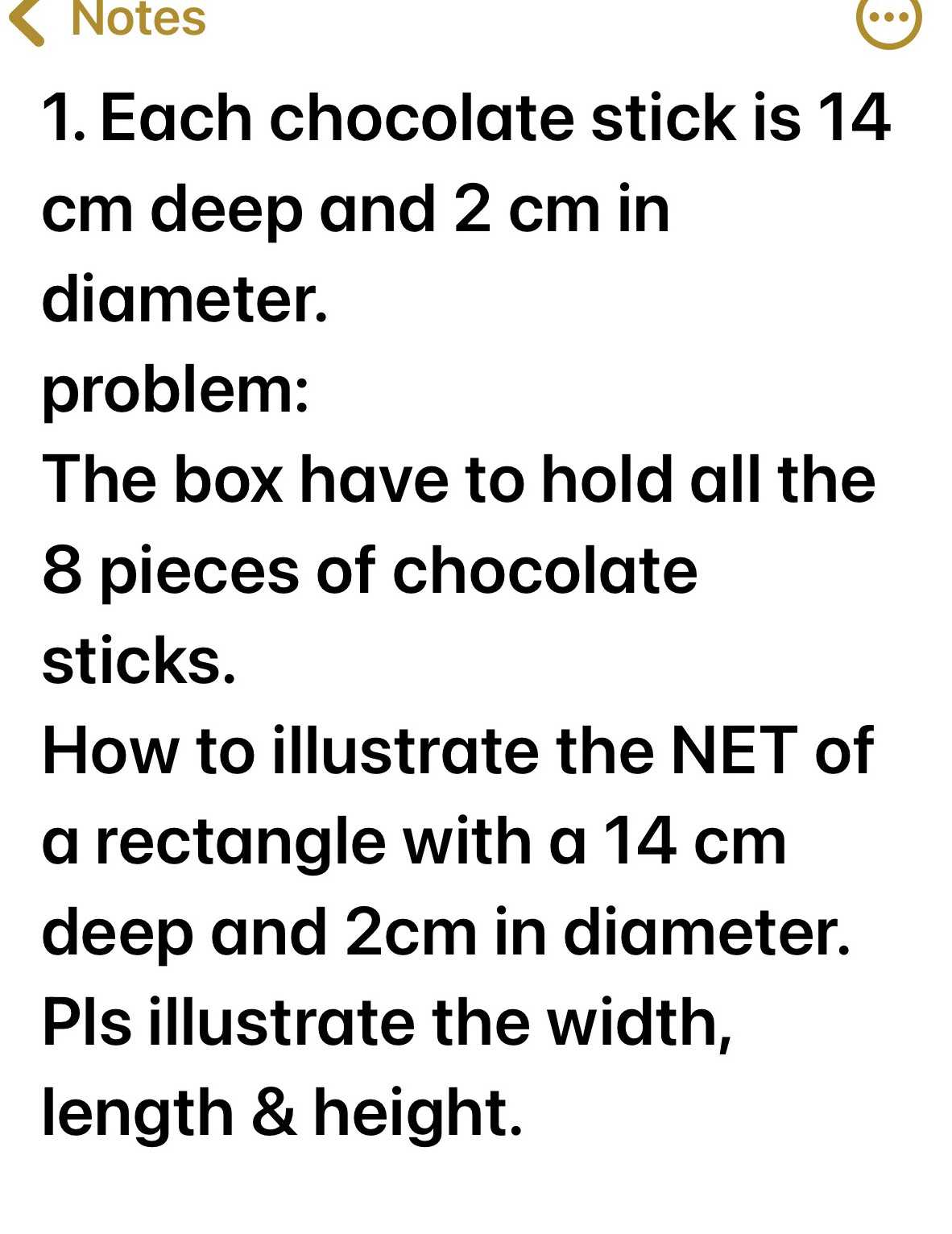### ¿Todavía tienes preguntas de matemáticas?

Pregunte a nuestros tutores expertos
Algebra
Pregunta1. Each chocolate stick is $$14$$ cm deep and $$2 cm$$ in diameter. problem: The box have to hold all the

$$8$$ pieces of chocolate sticks. How to illustrate the NET of a rectangle with a $$14 cm$$ deep and $$2 cm$$ in diameter. Pls illustrate the width, length \& height.